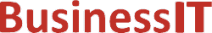O nás     Inzerce     KontaktSpolehlivé informace o IT již od roku 2011[Linux manuál]

# hypot, hypotf, hypotl: Euklidovská vzdálenostní funkce

Originální popis anglicky: hypot, hypotf, hypotl - Euclidean distance function

Návod, kniha: POSIX Programmer's Manual

## STRUČNĚ

#include <math.h>

double hypot(double x, double y );

float hypotf(float x, float y);

long double hypotl(long double x, long double y);

## POPIS / INSTRUKCE

These functions shall compute the value of the square root of x**2+ y**2 without undue overflow or underflow.
An application wishing to check for error situations should set errno to zero and call feclearexcept(FE_ALL_EXCEPT) before calling these functions. On return, if errno is non-zero or fetestexcept(FE_INVALID | FE_DIVBYZERO | FE_OVERFLOW | FE_UNDERFLOW) is non-zero, an error has occurred.

## NÁVRATOVÁ HODNOTA

Upon successful completion, these functions shall return the length of the hypotenuse of a right-angled triangle with sides of length x and y.
If the correct value would cause overflow, a range error shall occur and hypot(), hypotf(), and hypotl() shall return the value of the macro HUGE_VAL, HUGE_VALF, and HUGE_VALL, respectively.
If x or y is ±Inf, +Inf shall be returned (even if one of x or y is NaN).
If x or y is NaN, and the other is not ±Inf, a NaN shall be returned.
If both arguments are subnormal and the correct result is subnormal, a range error may occur and the correct result is returned.

## CHYBY / ERRORY

These functions shall fail if:
Range Error
The result overflows.
If the integer expression (math_errhandling & MATH_ERRNO) is non-zero, then errno shall be set to [ERANGE]. If the integer expression (math_errhandling & MATH_ERREXCEPT) is non-zero, then the overflow floating-point exception shall be raised.

These functions may fail if:
Range Error
The result underflows.
If the integer expression (math_errhandling & MATH_ERRNO) is non-zero, then errno shall be set to [ERANGE]. If the integer expression (math_errhandling & MATH_ERREXCEPT) is non-zero, then the underflow floating-point exception shall be raised.

The following sections are informative.

None.

## APPLICATION USAGE

hypot(x,y), hypot(y,x), and hypot( x, - y) are equivalent.
hypot(x, ±0) is equivalent to fabs(x).
Underflow only happens when both x and y are subnormal and the (inexact) result is also subnormal.
These functions take precautions against overflow during intermediate steps of the computation.
On error, the expressions (math_errhandling & MATH_ERRNO) and (math_errhandling & MATH_ERREXCEPT) are independent of each other, but at least one of them must be non-zero.

None.

None.

## SOUVISEJÍCÍ

feclearexcept() , fetestexcept() , isnan() , sqrt() , the Base Definitions volume of IEEE Std 1003.1-2001, Section 4.18, Treatment of Error Conditions for Mathematical Functions, <math.h> Portions of this text are reprinted and reproduced in electronic form from IEEE Std 1003.1, 2003 Edition, Standard for Information Technology -- Portable Operating System Interface (POSIX), The Open Group Base Specifications Issue 6, Copyright (C) 2001-2003 by the Institute of Electrical and Electronics Engineers, Inc and The Open Group. In the event of any discrepancy between this version and the original IEEE and The Open Group Standard, the original IEEE and The Open Group Standard is the referee document. The original Standard can be obtained online at http://www.opengroup.org/unix/online.html .
 2003 IEEE/The Open Group
©2011-2020 BusinessIT.cz, ISSN 1805-0522 | Názvy použité v textech mohou být ochrannými známkami příslušných vlastníků.
Provozovatel: Bispiral, s.r.o., kontakt: BusinessIT(at)Bispiral.com | Inzerce: Best Online Media, s.r.o., zuzana@online-media.cz
O vydavateli | Pravidla webu BusinessIT.cz a ochrana soukromí | pg(9121)